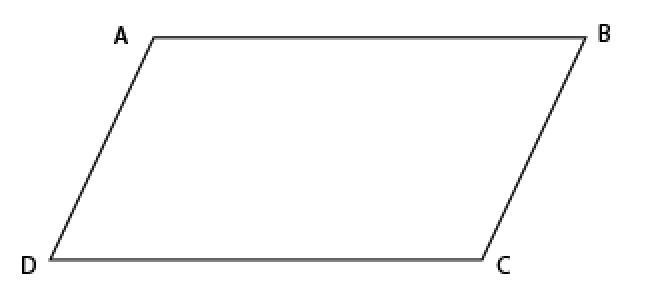# GMAT Question of the Day - Data Sufficiency - GeometryIs the perimeter of parallelogram ABCD greater than 10?

(1)  Sides AB and BC are in a ratio greater than 7 to 1
(2)  The distance from A to C is 5

## GMAT Question of the Day Solution:

(1) The sides being in a certain ratio doesn't tell us anything about the perimeter. AD could be 1000 and AB could be 7000 or AD could be .1 and AB could be .7. Insufficient.

(2) The diagonal is 5. Whenever there is a perimeter question dealing with triangles always think of the third side rule: The third side of a triangle must be greater than the difference and less than the sum of the other two sides. In this case AD + AB must be greater than 5. Same thing for DC + BC. So the perimeter must be greater than 10. Sufficient.

# CONTACT

Atlantic GMAT

405 East 51st St.

NY, NY 10022

(347) 669-3545

info@AtlanticGMAT.com